# High School Physics : Understanding Newton's Second Law

## Example Questions

### Example Question #1 : Newton's Laws

Which of the following is not a part of Newton's second law?

Acceleration

Mass

Displacement

Force

Displacement

Explanation:

Newton's 2nd law states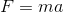. Therefore, all we need is a force, a mass, and an acceleration!

### Example Question #1 : Newton's Laws

A block is pushed withnewtons of force. What other information do we need in order to find the acceleration of the block?

Final velocity

Initial velocity

Mass

Work done on the block

Acceleration due to gravity

Mass

Explanation:

Newton's second law states that.

If we know the force,, then we only need to know the mass,, in order to find acceleration.

### Example Question #3 : Understanding Newton's Second Law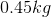orange falls from a tree. What is the force of gravity on the orange?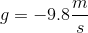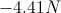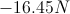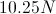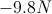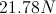Explanation:

Newton's second law states that:We are given the mass of the orange and the acceleration; since we are looking at the force due to gravity, the acceleration will be the acceleration due to gravity. Use these given values to calculate the force.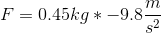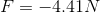Keep in mind that the force will be negative, since gravity acts in the downward direction.

### Example Question #1 : Understanding Newton's Second Law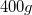ball rests on a flat table. What is the normal force exerted on the ball by the table?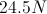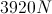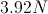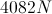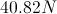Explanation:

Newton's second law allows us to solve for the force of gravity on the ball: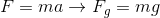Newton's third law tells us that the force of the ball on the table, due to gravity, will be equal and opposite to the normal force of the table on the ball.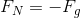Substitute the equation for force of gravity.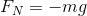Now we can use the mass of the ball and the acceleration of gravity to solve for the normal force. First, convert the mass to kilograms. Then, use the equation to find the normal force.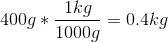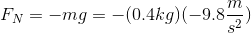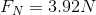### Example Question #1 : Understanding Newton's Second Law

Which of these is necessary for there to be a non-zero net force?

An equal and opposite reaction

Non-zero frictional force

Non-zero net torque

Non-zero net acceleration

Non-zero net displacement

Non-zero net acceleration

Explanation:

Newton's second law states that force is a mass times an acceleration.In order for a force to exist, there must be an acceleration applied to a mass. A force cannot exist on a massless object, nor can it exist without a net acceleration.

Newton's third law states that for every force on an object, there is an equal and opposite force from the object. These force frequently cancel out, however, and produce a net force of zero.

### Example Question #6 : Understanding Newton's Second Law

Which of these is not an example of Newtonian mechanics?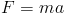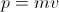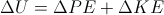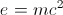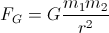Explanation:

Newtonian mechanics apply to all objects of substantial mass travelling at significantly slower than the speed of light.

Newton's law of universal gravitation, Newton's second law, momentum, and the equation for mechanical energy all fall under Newtonian mechanics.

The mass-energy equivalence suggests that mass can change as the speed of an object (such as an electron) approaches the speed of light. Newtonian mechanics assume that mass is constant, and do not apply to objects approaching the speed of light.

### All High School Physics Resources